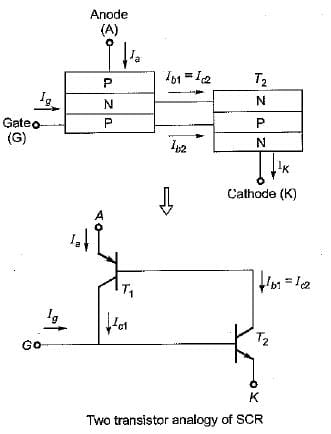# Test: Thyristors

## 10 Questions MCQ Test Topicwise Question Bank for Electrical Engineering | Test: Thyristors

Description
Attempt Test: Thyristors | 10 questions in 30 minutes | Mock test for Electrical Engineering (EE) preparation | Free important questions MCQ to study Topicwise Question Bank for Electrical Engineering for Electrical Engineering (EE) Exam | Download free PDF with solutions
QUESTION: 1

Solution:
QUESTION: 2

Solution:
QUESTION: 3

### Assertion (A) : The operation of an SCR can be explained in a very simple way by considering it in terms of two transistors. Reason (R) : in the two transistor analogy of the SCR, the SCR can be considered as an npn and a pnp transistor, where the collector of one transistor is attached to the emitter of the other and vice-versa.

Solution:

In the two transistor analogy of the SCR, the SCR can be considered as an npn and a pnp transistor, where the collector of one transistor is attached to the base of the other and vice-versa as shown below. Thus, reason is a false statement.QUESTION: 4

After firing an SCR, the gate pulse is removed. The current in the SCR will

Solution:

Once the thyristor (SCR) is turned-on, and the anode current is above latching current level, there is no need of gate current, hence, gate signal can be withdrawn i.e. gate looses control over the anode current.

QUESTION: 5

Thyristor is not made using germanium, because

Solution:

The leakage current in silicon is of the order of nA while in Germanium it is in μA.

QUESTION: 6

Thyristor is a semiconductor switch which is

Solution:

Like the diode, an SCR is an unidirectional device that blocks the current flow from cathode to anode i.e. unilateral. An SCR or a thyristor has three modes of operation namely forward conduction mode, forward blocking mode and reverse blocking mode. Out of these three modes of operation the first two are stable states due to which an SCR is bistable.

QUESTION: 7

Assertion (A) : If the one-state anode current drops below a minimum level, designated as the holding current IH, the thyristor reverts to the forward blocking state.
Reason (R) : The loop gain of the equivalent pnp-npn transistors fall below unity and the regenerative hold-on action ceases.

Solution:
QUESTION: 8

The main reason for connecting a pulse transformer at the output stage of a thyristor triggering circuit is to

Solution:

Pulse transformers are often used to couple a trigger pulse generator to a thyristor in order to obtain electrical isolation between the two circuits.

QUESTION: 9

Surge current rating of an SCR specifies the maximum

Solution:

The surge current rating indicates the maximum possible non-repetitive or surge current, the device can withstand and this may occur due to non-repetitive faults or short-circuits during the life-span of a thyristor.

QUESTION: 10

The drawback of R-firing circuit for SCR is

Solution:

The R-firing circuit suffers from several disadvantages. One of them is that the trigger angle can be varied only upto an approximate value of 90°.Use Code STAYHOME200 and get INR 200 additional OFF Use Coupon Code